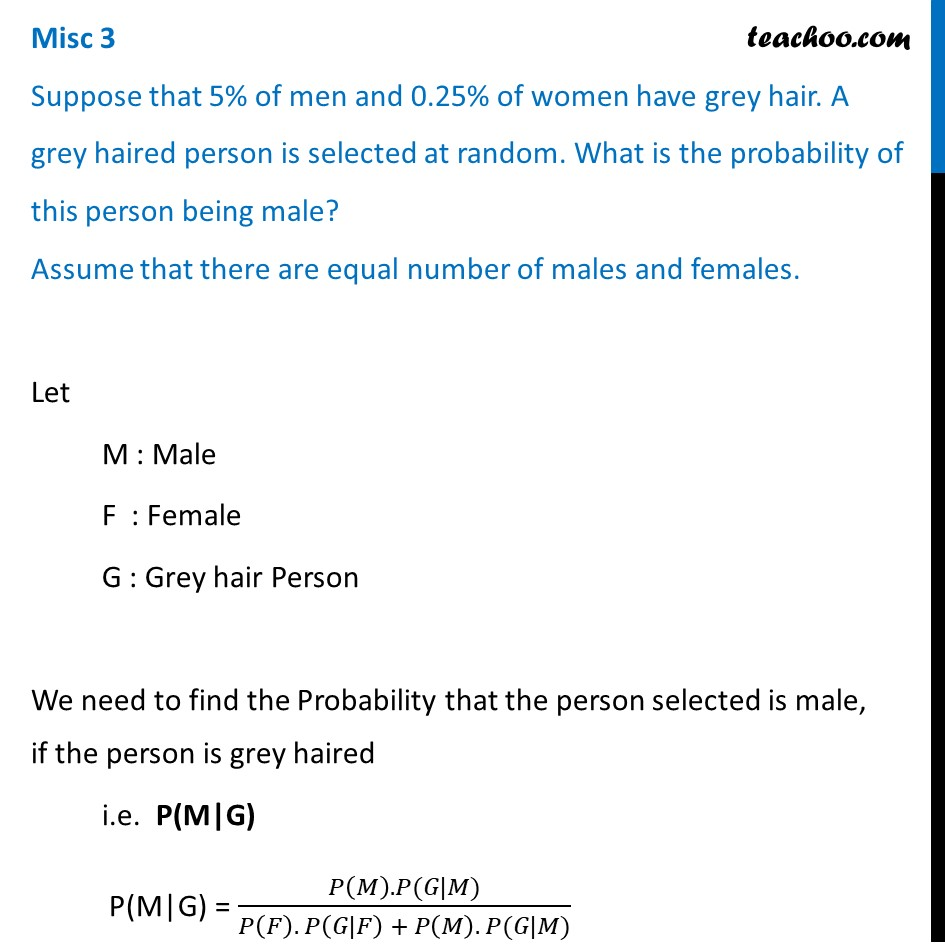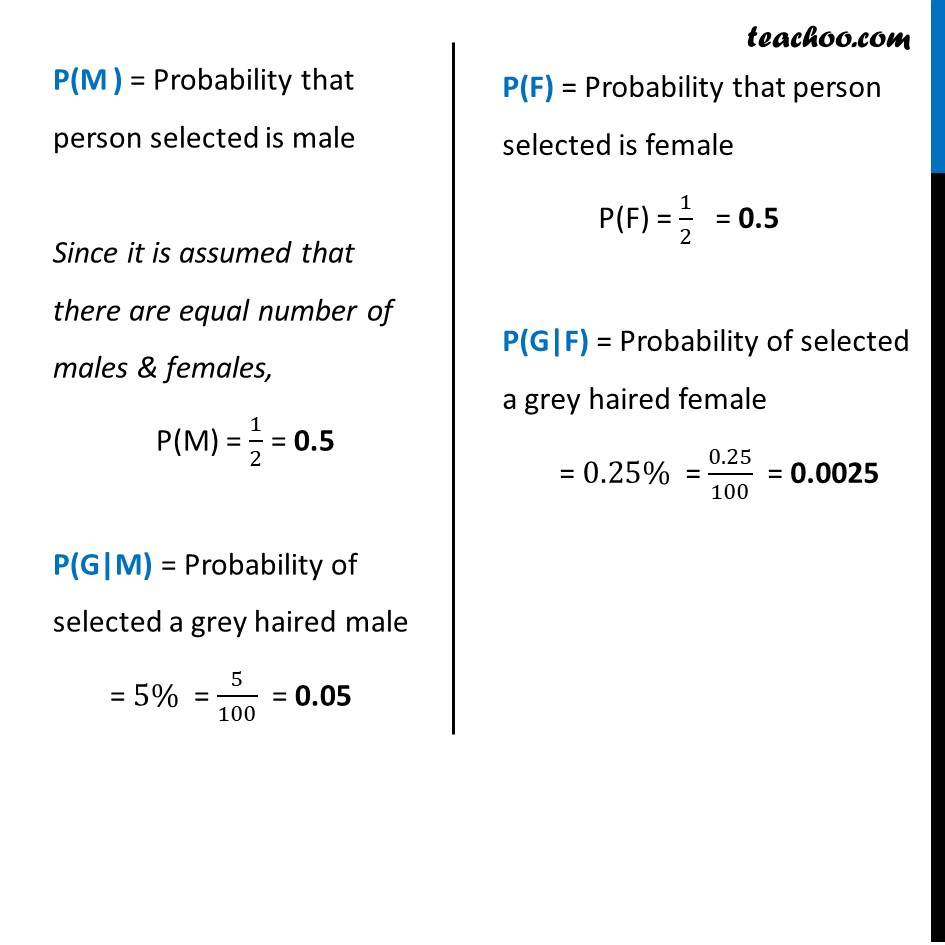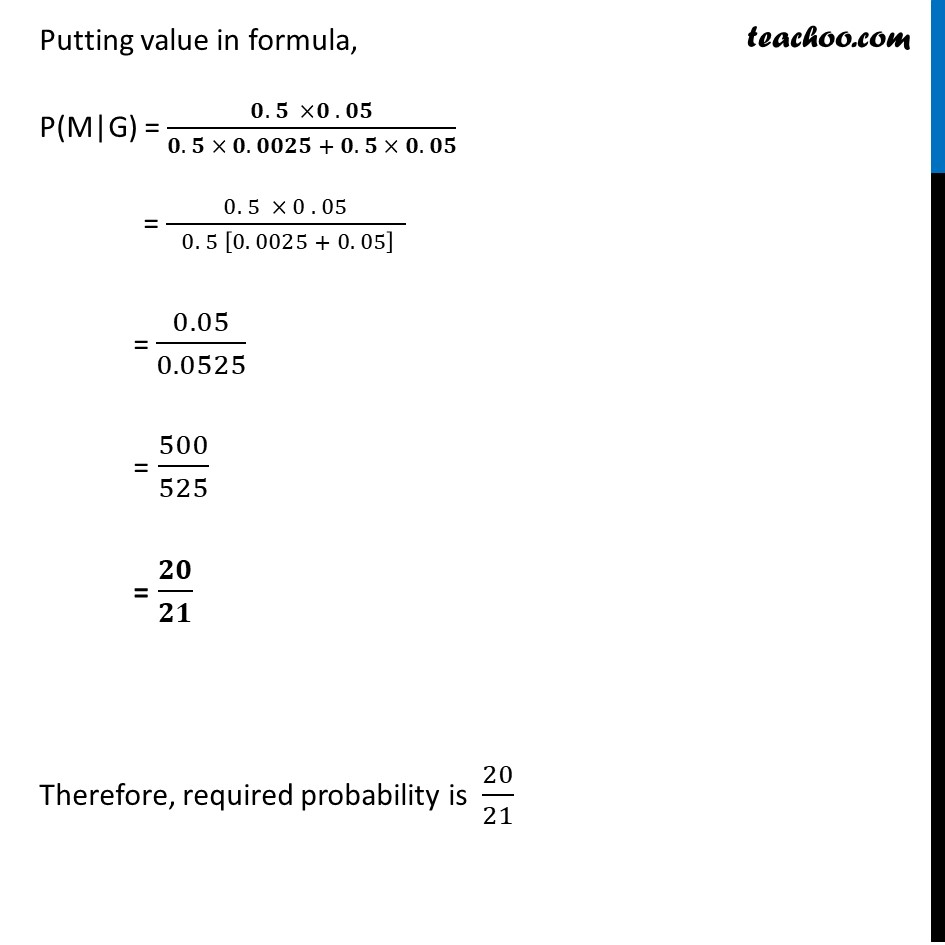Miscellaneous

Chapter 13 Class 12 Probability
Serial order wiseLearn in your speed, with individual attention - Teachoo Maths 1-on-1 Class

### Transcript

Misc 3 Suppose that 5% of men and 0.25% of women have grey hair. A grey haired person is selected at random. What is the probability of this person being male? Assume that there are equal number of males and females.Let M : Male F : Female G : Grey hair Person We need to find the Probability that the person selected is male, if the person is grey haired i.e. P(M"|"G) P(M"|"G) = (𝑃(𝑀).𝑃(𝐺|𝑀))/(𝑃(𝐹). 𝑃(𝐺|𝐹) + 𝑃(𝑀). 𝑃(𝐺|𝑀)) "P(M )" = Probability that person selected is male Since it is assumed that there are equal number of males & females, "P(M)" = 1/2 = 0.5 "P(G|M) "= Probability of selected a grey haired male = 5% = 5/100 = 0.05 "P(F)" = Probability that person selected is female "P(F)" = 1/2 = 0.5 "P(G|F) "= Probability of selected a grey haired female = 0.25% = 0.25/100 = 0.0025 Putting value in formula, "P(M|G) "= (𝟎. 𝟓 ×𝟎 . 𝟎𝟓)/(𝟎. 𝟓 × 𝟎. 𝟎𝟎𝟐𝟓 + 𝟎. 𝟓 × 𝟎. 𝟎𝟓) = (0. 5 × 0 . 05)/( 0. 5 [0. 0025 + 0. 05] ) = 0.05/0.0525 = 500/525 = 𝟐𝟎/𝟐𝟏 Therefore, required probability is 20/21